# Electronic Filters

## 1 OBJECTIVES

The experimental objective of this lab is to build two electrical circuits and to test them using NI-ELVISmx instruments in order to identify each as a low-pass, or high-pass, or band-pass filter. The -3dB point for each filter will also be identified. Finally, we'll build a simple radio that doesn't require a battery to operate as an example of a band-pass filter.

Together, we will learn how electrical filters are used, how resistors, capacitors, and inductors function in a filter and the significance of the -3dB point.

## 2 OVERVIEW

It is important for electrical engineers to be able to selectively filter certain frequencies. An electronic filter works by allowing only designated frequencies to pass through. By tuning your radio to a particular station, you are isolating a certain frequency. The filter selects the station you have chosen from the hundreds of different stations that are broadcasting. How does the filter accomplish this?

To understand how filters work, we need to identify the elements that make electrical filtering possible. Signals that occur naturally are composed of many frequencies.

For example, the human voice is composed of frequencies ranging from 0–4kHz. A signal consists of a primary frequency, called a base frequency, plus additional multiples of that frequency with different loudness called harmonics. Different devices will have different harmonics. This is why when a trumpet and clarinet both play the same musical note they sound different — their harmonics are different. We hear the base frequency plus the harmonics of these instruments. Rock guitars have their distinctive sound by adding harmonics to the note they play.

Radio waves work differently than sound waves. The signal has a base frequency, and the information we hear is contained in adjacent frequencies called sidebands.

Voltage, or electromotive force, and frequency are the building blocks of an electrical signal. Voltage is a force that propels electrons through a medium. Current is the amount of electric charge flowing past a specified circuit point per unit time. Frequency is the rate at which the signal repeats itself. The gain of a circuit is the ratio of its output power compared to its input. For devices that do not produce energy, such as this lab, the gain cannot exceed one. However for devices such as transistors and other amplifiers, the gain can be much greater than one.

A filter is a circuit that shapes and controls the bandwidth of a signal; bandwidth is the range of frequencies that the filter allows to pass. For example, when we turn up the bass of an audio amplifier, we are using a filter that passes the low frequencies more than the high frequencies. Also, when we tune a radio, we are using a filter that allows the base frequency plus the sidebands of the desired station to pass, but not other stations.

The filters that we are going to build are composed of resistors and capacitors. A resistor resists the flow of electrons by converting some of the electric energy to heat. The voltage across a resistor obeys Ohm's Law: V = IR, where I is the current and R is the resistance. Varying the frequency of the voltage will not affect the voltage across the resistor.

A resistor is designed to impede the flow of electricity and dissipate electrical energy in the form of heat. Resistors work by having the electricity flow through a poor conductor such as carbon. The unit of resistance is the Ohm, named after Georg Ohm, and is represented by the capital Greek letter Omega ("Ω").

Capacitors are metal plates that are separated from each other, allowing electric charge to accumulate on the plates. These plates store energy rather than dissipate it, like a resistor. For DC voltage, provided by a device like a battery, the plates will gather charge, and once the plates are charged, will no longer pass current, so the capacitor looks like an "open circuit", where nothing is connected. For high frequencies, the charge can quickly gather and dissipate off the plates, allowing current to flow through the capacitor freely, making the capacitor behave like a "short circuit". The unit of capacitance is the Farad, named after Michael Faraday, and is represented by the capital letter "F". The relationship between the voltage across a capacitor and varying frequencies is shown in Figure 1.

Inductors, also known as coils or chokes, are coils of wire that allow the current through the wire to form a magnetic field. Like capacitors, inductors store energy, but in the magnetic field instead of on plates, and its behavior is the opposite of a capacitor. For DC voltage, the current will make a stable magnetic field and the current will flow freely, making the inductor look like a "short circuit". At high frequencies, the magnetic field does not have time to form before the current reverses and the field collapses, causing the inductor to resist the current flow, looking like an "open circuit". The unit of inductance is the Henry, named after Joseph Henry, and is represented by the capital letter "H". The relationship between the voltage across an inductor and varying frequencies is shown in Figure 2.

The elements of filters, such as resistors, capacitors, and inductors, are connected by conductors (wires) that carry electrons between these devices. The voltage is the same throughout the entire conductor, so the voltage across the two ends of the conductor is zero.

How the components are connected is shown using a schematic diagram. Each component is represented by a symbol, and the connections between components are represented by lines. The symbols are designed to represent the physical characteristics of the components. Therefore, a resistor is represented by a zigzag line showing how the electricity's path is impaired. A capacitor is shown as two parallel lines, representing its plates. Finally, an inductor is shown as a series of looping lines, representing the coils of wire it has. The schematic diagram symbols for these components are shown in Figure 3.

Different combinations of resistors and capacitors allow engineers to build different kinds of filters to perform specific tasks. Resistors, inductors, and capacitors can be arranged in three different ways. In a series circuit, the element's conductors are connected end to end. The current in a series circuit remains the same in all the electrical elements. In a series circuit, as shown in Figure 4, the sum of the voltages across each element is equal to the voltage of the power source (VIN = VA + VB + VC).

In a parallel circuit, as shown in Figure 5, the element's conductors are connected at opposing ends. The current that is supplied by the voltage source equals the current that flows though elements D and E. The voltage across the elements that are parallel is the same (VIN = VD = VE)).

Series and parallel circuits can be combined, as shown in Figure 6:

The voltage across the top and bottom connectors is the same, so (VIN = VF = VG = (VH + VJ)).

To produce a graphical representation of the characteristic behavior of the circuit being analyzed, it is necessary to graph the gain of the circuit versus the frequency of the electrical signal. Gain is a measure of the power produced by the circuit, measured in decibels, and is calculated using the following formula:A 3dB drop of signal power is the point at which the signal power is half of its original value. The frequency corresponding to this -3dB point is also called the cutoff frequency of the filter. Note that since the filters we are using only contain passive components, the output cannot be larger than the input. Therefore, the gain will always be a negative number because we are taking the logarithm of a number less than one.

If you examine the preceding formula, you'll see that for the gain to be -3dB, VOUT must be VIN divided by the square root of 2 (1.414), more easily written as VOUT=VIN/1.414. Therefore, the -3dB point of a circuit is the frequency that causes the output voltage to be .707 times the input voltage.

### Types of Filters

Your job, once you have built the electrical filters according to the specifications in this manual, is to identify them. In order to do this, we must define the three possibilities.

Sometimes, it is beneficial to remove the high frequency components from a signal because this is where unwanted noise frequently exists. To do this, a low-pass filter is used. It passes the low frequencies while blocking the higher ones. The low-pass filter shown here has a gain that drops from 0dB to -3dB at 1590 Hz, which is its -3dB point and corresponding cut-off frequency. Thus, the filter has a bandwidth of 1590Hz and it only allows frequencies from 0Hz to 1590Hz to pass through at full power. Figure 7 shows the characteristic behavior of a low-pass filter. A trend line showing the response of the filter is also shown. Note that for low frequencies, the gain is 0dB, meaning that the output equals the input. The filter suppresses high frequencies, as shown by the trend line on the right. The intersection of these two trend lines will be at the cutoff frequency, giving another reason why the cutoff frequency is important.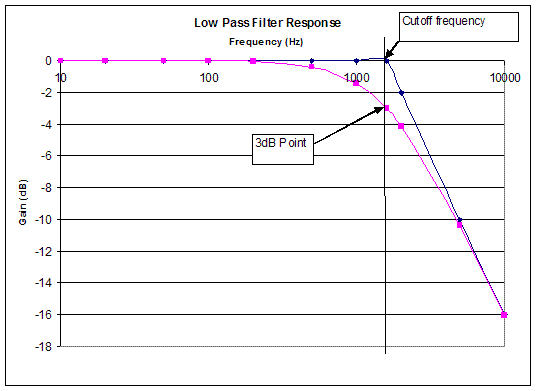Figure 7: Characteristic behavior of a low-pass filter

A high-pass filter passes the high frequencies but blocks the low ones, the opposite response of a low-pass filter. The -3dB point for this filter is 160 Hz. This is determined using the same method we used for the low-pass filter. Figure 8 shows the characteristic behavior of a high-pass filter as well as the trend lines and their intersection at the cutoff frequency. Thus, we can say that the filter has a bandwidth of 160 Hz to infinity and that the filter only allows frequencies greater than 160 Hz to pass through.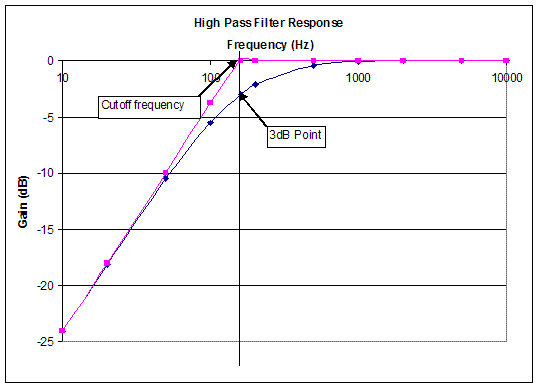Figure 8: Characteristic behavior of a high-pass filter

A band-pass filter only allows a certain range of frequencies to pass through and blocks all other frequencies. The frequency of the highest response point is called the resonant frequency, and for an ideal filter will be 0dB. This type of filter has two -3dB points, one above the resonant frequency and one below it, and therefore two cutoff frequencies. The difference in these two cutoff frequencies is called the bandwidth of the band-pass filter. Figure 9 shows the characteristic behavior of a band-pass filter.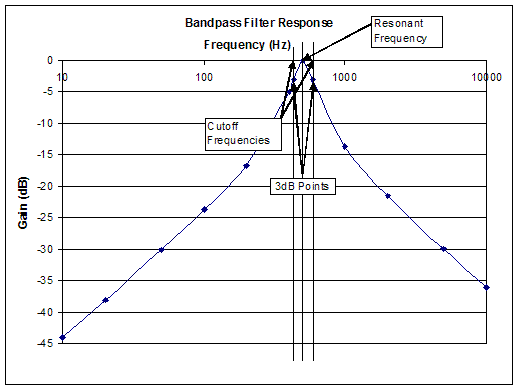Figure 9: Characteristic behavior of a band-pass filter

The band-pass filter shown here has a resonant frequency of approximately 500Hz, and has -3dB points with cutoff frequencies of approximately 400Hz and 600Hz. Therefore, the bandwidth of this filter is approximately 600Hz-400Hz, or 200Hz.

Finally, a notch filter rejects a specific range of frequencies and passes the others. Looking at it another way, a notch filter is the exact opposite of a bandpass filter. Notch filters are frequently used to remove interference from a desired signal.

Note: the filters here are "ideal" filters, and show total power transfer (0dB) at their maximum response. Real filters frequently show some losses, and may have a maximum response less than 0dB. However, the -3dB point is still defined according to the formula shown above.

Now, we are ready to build the circuits that will produce the electronic filters. Before we start, it is important to understand the breadboard we will be using to connect the elements of our circuits. Breadboards have horizontal and vertical connectors. The front of the breadboard is shown in Figure 10a. The back of the breadboard, with its protective cover removed so you can see the internal connections, is shown in Figure 10b.

(Red squares indicate where connections are broken, making two independent halves)

(Red squares indicate where connections are broken, making two independent halves)

Finally, you'll need to identify the resistors and the capacitors required to complete your circuit. To identify the resistors, you must look at the colored bands. Hold the resistor horizontally with the three bands that are close together to the left, as shown in Figure 11. The first two colors determine the first two digits of the resistance, and the third band determines how many zeroes to add. Each colored band represents a particular number.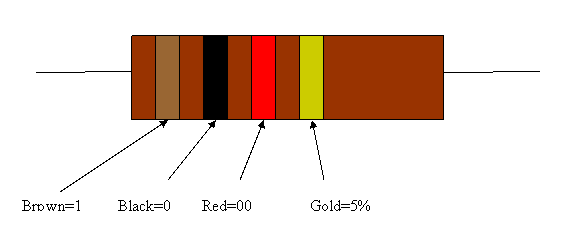Figure 11: Color-coded bands on a 1kΩ resistor

Resistor color code:

 Color First Band Second Band Third Band Black 0 0 X1 Brown 1 1 X10 Red 2 2 X100 Orange 3 3 X1000 Yellow 4 4 X10000 Green 5 5 X100000 Blue 6 6 X106 Violet 7 7 X107 Gray 8 8 X108 White 9 9 X109

The fourth band indicates a tolerance of the resistor, which means how close its actual resistance will be to the value indicated by the bands:

 Color Tolerance Gold 5% Silver 10% No band 20%

As an example, a 470kΩ resistor with a 5% tolerance would have color bands of yellow, violet, yellow, and gold. You should check this yourself to be sure you know how to read the colors.

For capacitors, the value is stamped on the capacitor, especially if the capacitor is large. Most capacitors have very small values. For small capacitors, the most common ranges are microfarads (shown as µF), nanofarads (shown as nF), and picofarads (shown as pF). The following conversion table may help you understand how these ranges relate to each other:

1 µF=103nF=106F
1 nF=103pF

Sometimes the value is coded. The following rules are commonly used in marking values on capacitors:

• If there are only two digits on the capacitor, the value is in picofarads (nF)
• For three digits, the first two digits of the number are the overall value of the capacitor, and the third is a power of 10, with the overall value being in picofarads. For example, a capacitor with a value of 103 would be 10*103 picofarards, or .01 nanofarads. To make this a little easier, the following table might be helpful:

 CODE/Marking µFmicrofarads nFnanofarads pFpicofarads 100 0.00001 0.01 10 101 0.0001 0.1 100 102 0.001 1 1000 103 0.01 10 10000 104 0.1 100 100000 105 1 1,000 106 106 10 10,000 107 107 100 100000 108
• Finally, capacitors might use a series of colored bands, like resistors, with exactly the same meaning, except the units are in picofarads instead of ohms.

Also, where there are digits on the capacitor, there might be a letter following the digits. This indicates the tolerance of the capacitor according to the following table:

 C +/-0.25pF D +/-0.5pF F 1% G 2% J 5% K 10% M 20% Z +80-20%

The following figures show two capacitors. You can use the preceding tables to verify their values.

For inductors, the value is stamped on the inductor. If you can't find the value, ask your TA for help.

## 3 YOUR ASSIGNMENT

### Individual Lab Report

Follow the lab report guidelines laid out in the page called Specifications for Writing Your Lab Reports in the Technical Communication section of this manual. As you write, the following discussion points should be addressed in the appropriate section of your lab report:

• What is an oscilloscope? You may have to do some research to answer this question.
• Discuss the different types of electrical elements. (i.e. resistor, capacitor, inductor)
• Observe the graphs generated from each circuit. What type of filter does each circuit produce?
• Does each circuit produce the expected result? If not, explain why.
• Locate the -3dB point on each graph and cutoff frequency to determine the bandwidth of each filter.
• What is the -3dB point used for?
• Name the three different types of filters and applications for each.
• Discuss any problems encountered, was the experiment a success or a failure? If a failure, why?
• Describe the operation/circuitry of the crystal set.

### Team PowerPoint Presentation

Follow the presentation guidelines laid out in the page called EG1003 Lab Presentation Format in the Introduction to Technical Presentations section of this manual.When you are preparing your presentation, consider the following points:

• How do the results obtained validate the function of the filters you created?
• What are some applications of electronic filters?
• Why is the -3 dB point important? What is its significance? How is it determined?

## 4 MATERIALS AND EQUIPMENT

• 2 100kΩ Resistors
• 1MΩ Resistor
• 0.001µF Capacitor
• 0.01µF Capacitor
• NI-ELVIS II+ with Prototyping Board
• Coaxial cables with alligator clips on one end
• 1N34 diode
• High impedance headphone
• 10pF capacitor
• 1mH inductor
• Appropriate Wiring

Remember: You are required to take notes. Experimental details are easily forgotten unless written down. EG Standard Note Paper can be downloaded and printed from the EG Website. Use your lab notes to write the Procedure section of your lab report. At the end of each lab your TA will scan your lab notes and upload them to the Lab Documents section of the EG Website. You must attach your lab notes at the end of your lab report (use the "Insert Object" command in MS Word after your Conclusion). Keeping careful notes is an essential component of all scientific practice.

## 5 PROCEDURE

### Circuit Testing

1. Plug in the NI-ELVIS II+ and connect the USB cable to the Lab PC.
2. Power on the NI-ELVIS II+ by toggling the switch on the back of the device. When the pop-up window appears, select NI-ELVISmx Instrument Launcher and click OK.
3. Select FGEN (the function generator) in the Instrument Launcher window.
4.Figure 14: NI ELVISmx Instrument Launcher window.
Set the frequency to 1000Hz in the Function Generator window. Alternatively, you can also type 1000 in the box. Also, set the amplitude to 2 Vpp (Volts peak-to-peak) and set the signal route to FGEN BNC. Make sure that the sine wave is selected on the left.
5. Select Scope (the oscilloscope) in the Instrument Launcher window.
6. Connect one coaxial cable to FGEN and another one to CH 0 on the NI-ELVIS II+ (located at the upper left corner of the device).
7. Clip the red and black alligator clips together.
8. Click run in the Function Generator and Oscilloscope windows.
9. Make sure the oscilloscope on your lab PC looks similar to Figure 15. If the wave appears as if it is horizontally compressed, change the Timebase setting until the waveform looks similar to that of the figure.
10. Record the Vp-p reading. This is your VIN. When you have finished calibrating the function generator and the oscilloscope, the Vp-p (or Vpp) reading on your lab PC will be approximately 2.000V. Use this figure for all your computations. Also, calculate .707*VIN and write it down. This is your -3dB point voltage for finding the cutoff frequency.
11. Now we will build Circuit 1. Stop the function generator. DO NOT close the program! Doing so will result in losing the settings that were configured. Use the schematic diagram in Figure 16 to build your circuit.
12.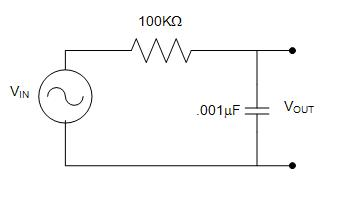Figure 16: Circuit 1 (R = 100kΩ, C = 0.001µF)
13. Insert one end of a 100kΩ resistor into the breadboard at B3 and the other end at G3.
14. Insert one end of a 0.001μF capacitor into the breadboard at H3 and the other end at H10.
15. Attach the red alligator clip from the CH 0 cable to the top lead of the capacitor, i.e. the one connected with the resistor. Connect the black alligator clip to the bottom lead of the capacitor, i.e. the one not connected to the resistor.
16. Affix the red alligator clip from the FGEN cable to left end of the resistor, i.e. the one not connected to the capacitor. Connect the black alligator clip to the bottom lead of the capacitor, i.e. the one not connected to the resistor, and that also has an alligator clip connected to it from the CH 0 cable.
17. Run the function generator. Change the frequency on the function generator in this pattern (10, 20, 50, 100, 200, 500, 1000, 2000, 5000, 10000Hz) and record the Vp-p reading at each frequency for a total of 10 measurements. Looking at your results, you will see voltages above and below the -3dB point voltage. Now find the -3dB point exactly by starting with the frequency just below the -3dB point, and then increasing the frequency until you have the voltage corresponding to the -3dB point. Note the frequency and write it down. This is the cutoff frequency. If the frequency reading on the oscilloscope is a question mark, or the Vp-p reading fluctuates significantly, adjust the Scale Volts/Div and/or the Time/Div setting until the frequency reading gives you a number.
18. Stop the function generator and disconnect all the wires, capacitors, and resistors from the breadboard. Use the schematic diagram in Figure 17 to build Circuit 2.
19.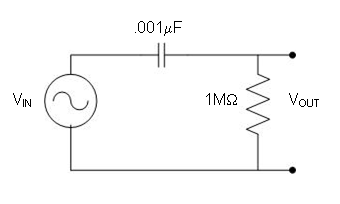Figure 17: Circuit 2 (R = 1 MΩ, C = 0.001µF).
20. Connect the red alligator clip from the CH 0 cable to the top lead of the resistor, i.e. the one connected with the capacitor. Connect the black alligator clip to the bottom lead of the resistor, i.e. the one not connected to the capacitor.
21. Attach the red alligator clip from the FGEN cable to left end of the capacitor, i.e. the one not connected to the resistor. Connect the black alligator clip to the bottom lead of the resistor, i.e. the one not connected to the capacitor, and that also has an alligator clip connected to it from the CH 0 cable. If you have wired your circuit successfully, the oscilloscope will have a horizontal line across the center of the screen.
22. Run the function generator. Change the frequency on the function generator in this pattern (10, 20, 50, 100, 200, 500, 1000, 2000, 5000, 10000Hz) and record the Vp-p reading at each frequency for a total of 10 measurements. Looking at your results, you will see voltages above and below the -3dB point voltage. Now find the -3dB point exactly by starting with the frequency just below the -3dB point, and then increasing the frequency until you have the voltage corresponding to the -3dB point. Note the frequency and write it down. This is the cutoff frequency.
23. Stop the function generator and disconnect the all the wires, capacitors, and resistors from the breadboard. Ask your lab TA for a small breadboard and use the schematic diagram in Figure 18 to build Circuit 3.
24. There are several items on the circuit diagram below that you might not recognize. The item in the top left corner that looks like a rake is an antenna, and will be connected after you've completed the rest of the circuit. Similarly, the item in the bottom left corner that has three horizontal lines is an "earth ground", which will also be connected later. Finally the component in the top middle that looks like an arrow going into a line is called a diode. You will be given a diode as part of building your circuit. What the diode does is beyond the scope of this lab, but you'll need it.

Circuit 3 is called a "crystal set" radio. As you can see, it is an actual working radio that doesn't require a battery. It got its name because originally a galena crystal was used instead of the 1N34 diode shown in the circuit diagram. As you can see, the symbol for a diode is an arrow pointing at a line. The band-pass filter is the inductor and capacitor shown on the left side of the circuit diagram. Since this design emphasizes quick assembly using a breadboard, you'll find that the radio can only get one or two stations, but it still works. More refined designs can the found on the World Wide Web via a search on the term "crystal set". A more detailed description of how the radio works is outside the scope of this lab, but a brief version is given in the Appendix below in case you are interested.

Note: To avoid confusion, only use the left half of the breadboard, i.e. the columns numbered 1 through 31.

25. Insert each end of a 1N34 (or 1N34A) diode into the breadboard horizontally (long direction) in one of the rows A through E.
26. Note: The orientation of the diode is not critical to the operation of the radio - it can be put in the circuit in either orientation.

27. Insert the 10pF capacitor into the breadboard vertically. Make sure that one of the two capacitor leads is inserted into the breadboard in the same column as one of the two leads of your diode.
28. Insert the second lead of your capacitor vertically in any pin in rows Y or Z.
29. Insert the 1mH inductor into the breadboard vertically in the same column as the diode and capacitor.
30. Insert the second lead of your inductor vertically in any pin in the same row (Y or Z) as the other end of the capacitor.
31. Note: You should now have three wires in one column of the breadboard. This is also where you will connect the antenna later, so remember this column. Similarly, the other ends of the inductor and capacitor should be in the same row. This is where you will connect the ground wire and one of the headphone wires later, so remember this row.

32. Insert one end of the 100kΩ resistor in the same column as the other end of the diode.
33. Insert the other end of the resistor into the same row (Y or Z) as the ends of the inductor and capacitor.
34. Connect one wire from the headphone to the same column as one end of the diode and resistor.
35. Connect the other headphone wire to the row that has the ends of the inductor, capacitor, and resistor.
36. Note: Once again, this row is where you'll attach the ground wire in a few minutes.

37. For this lab, we have created an AM transmitter (shown in Figure below) for you to use, which is located at the front desk in the lab room. Therefore, after you've assembled the rest of the radio, go to the transmitter and find the antenna, which is the green wire that has one exposed end. Connect it to your radio in the column we told you to remember earlier. It's the column that has one end of the inductor, capacitor, and diode.
38. Next, find the ground wire, which is the white wire with the exposed end. Connect that to your radio in the row (Y or Z) that we told you to remember earlier. This row contains one end of the inductor, capacitor, resistor, and headphone.
39. Put the headphone in your ear, and you should hear music. In your lab report and presentation, indicate quality and loudness of the music you heard from your crystal set.
40. After you've operated the radio, disconnect it from the antenna and ground, and return to the lab so you can put away the equipment and clean up your work area.

### Data Analysis

1. Create two tables in Microsoft Excel (one each for the low-and high-pass filter) to record your data. Remember your VIN is a constant. Each table should look like Figure 19.
2.  Frequency (Hz) VIN (Volts) VOUT (Volts) Gain=20log(VOUT/VIN) (dB) 10 2.0 20 2.0 50 2.0 100 2.0 200 2.0 500 2.0 1000 2.0 2000 2.0 5000 2.0 10000 2.0
Figure 19: Sample data table
3. Instruct Microsoft Excel to calculate the gain using this equation:
4.5. Plot two Gain(Y) vs. Frequency (X) graphs using the X, Y Scatter template. Make one graph for each circuit.
6. You'll find the template on the Insert tab, under the Charts group, with the Scatter icon. Click on the arrow below the icon and select the top left icon in the pulldown gallery. You can get the axes you want by clicking on the axis. You can get labels and titles by clicking on the chart, which will change the ribbon to the Design tab. In the Charts Layout group, click the icon that looks like the chart you want. Right click on the things you want to change.

7. Change the x-axis to logarithmic scale.
8. Right click on the x-axis and click on the Format axis item. For the window that pops up, about 1/3 down the window there's an item Logarighmic scale. Click the check box next to this item, and click Close.

9. Identify each of the circuits as a low-, high-, or band-pass filter. If it's a high-pass or low-pass filter, find the -3dB point and the cutoff frequency. If it's a band-pass filter, identify the two cutoff frequencies.
10. For a band-pass circuit, use the graph to find the -3dB point by seeing where the curve has a value of -3dB. Compare the value from the graph to the value you actually read earlier. Are they the same?

Your lab work is now complete. Please clean up your workstation. Return all unused materials to your TA. Refer to section 3 Your Assignment for the instructions you need to prepare your lab report.

## Appendix - How a Crystal Set Works

Note: This material is included for those who want to more about how a crystal set works. You do not have to read this section if you don't want to, and you will not be responsible for knowing this material

### History

Some of the earliest radios made were crystal sets. They have a number of advantages:

1. They do not require a power source such a a battery, so they can be used any time. This makes them very valuable during things like blackouts and recovery from disasters such as hurricanes.
2. They have so few components they can be built quickly, usually out of whatever parts are lying around
3. They are rugged because of their simplicity, so they can be used while you're traveling
4. Unlike regular radios, they do not emit a signal themselves, making them excellent "stealth" radios. This is important to people like soldiers, where they can listen to the radio without giving away their position.

The radio has several major components:

1. An antenna, which should be as long as possible - the longer the antenna, the more stations you'll hear.
2. An earth ground. As the name implies, this connects the radio to the earth, allowing an signals it receives to return to ground to complete the circuit for the voltage the signal makes.
3. A bandpass filter consisting of an inductor and a capacitor. Usually the values of one or both these components can be changed, or varied. For the inductor, if it can be varied it's by turning a rod going through the middle of the inductor. For the capacitor, if it can be varied the capacitor plates are visible, and can be rotated. Changing these values allows the allowable frequency to be changed, tuning the radio.
4. A diode, shown in the middle of the circuit diagram. If you could look at the signal from the bandpass filter, would look like a sine function (like you saw with the low-and high-pass filters), but instead of its amplitdue being constant, it would change substantially. A typical signal is shown below:

The variation in the waveform is the audio signal (sound) we want to hear. This is called Amplitude Modulation, or AM for short.

A diode is a device that passes electrical current in one direction. A galena crystal can be used as a diode, which is how the crystal set got its name. However, there are many other materials that can be used as a diode as well. During World War II, many soldiers used a rusty razor blade as a diode. After the invention of semiconductors, diodes are now made of silicon or germanium. For a crystal set, germanium is a better choice. In electronics in the United States, diodes have names that start with "1N", followed by a number and possibly a letter. The number is a serial number assigned by agreement among the electronics manufacturers. A letter, if present, indicates an improved version of the product. Therefore, the diode used here is 1N34, but the improved 1N34A could be used as well. Transistors always start with the designation of "2N", with other semiconductors have designations of "3N", "4N", and so on.

The diode is used as a detector, which detects the sound from the radio wave. It does this by removing the negative part of the sine wave, leaving only positive voltages. The headphones then act as a low pass filter, seeing only the height of the sine wave rather than the voltage variations of the sine wave itself. As we said earlier, this amplitude is tha audio signal we're looking for, so we hear it.

5. The headphone, which is how we hear the radio station. Sometimes a resistor is put in parallel with the headphone to boost the signal.

The negative thing about crystal sets is that they are essentially a craft item. Sometimes they don't work for no apparent reason. Sometimes they work in ways we don't totally understand. Many people view this as fun, not knowing what to expect. Others view it as a source of frustration. Either way, they are always exciting.

If you want to know more about crystal sets, the easiest way to get started is to use a search engine such as Google with a criterion such as "radio crystal set".